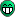# Convolution of two TF1

Dear all,

I need to perform a convolution of two TF1 I have created from a .txt data file. By creating a test (discret) convolution algorithm by myself I get the following plot (the result of the convolution of the pink one with itself is the black one):

I assume the black curve as the true curve for the convolution. Apparently, there is a class TF1Convolution.h which is supposed to give the same result. However, the result is completely different and I have no idea why. Here is the code I am using:

``````
// c++ includes
#include <iostream>
#include <stdio.h>
#include <fstream>

// root includes
#include "TROOT.h"
#include "TRandom3.h"
#include "TTree.h"
#include "TProfile.h"
#include <TMath.h>
#include <TString.h>
#include <TFile.h>
#include <TCanvas.h>
#include <TGraph.h>
#include <TAxis.h>
#include <TF1.h>
#include <vector>
#include "TVectorD.h"
#include "TSystem.h"
#include "TLatex.h"
#include <TF1Convolution.h>

using namespace std;

// TGraph to TF1
TGraph *g1;
double myfunc(double *xx, double *)
{
return g1->Eval(xx);
}

// Main function

void diego()
{
// Opening data. Here energy and PAI cross section relative to Rutherford cross section is provided
TTree *MyTree = new TTree("MyTree","MyTree");
Double_t energy, crossSection;

// Probability of energy deposition
Int_t nn = MyTree->GetEntries(); // number of entries on .txt file
const Int_t n = nn;
TVectorD ene(n), Pn1(n), sigma(n), Sigma(n);
TVectorD dSigma_dE(n), dSigmaRuth_dE(n), dSigmaRuth(n);

const Double_t beta_lorentz = 0.97;
const Double_t betagamma = beta_lorentz/sqrt(1 - pow(beta_lorentz,2));
const Double_t mc2 = 0.510998928*pow(10,6);
const Double_t Emax = 2*mc2*pow(betagamma,2);
const Double_t kr = 2.54955*pow(10,-19); // constant used in Rutherford cross-section (eV.cm2)

for (int i1=0; i1<n; i1++)
{
MyTree->GetEntry(i1);
ene(i1) = energy;
sigma(i1) = crossSection;

// Rutherford cross-section
dSigmaRuth_dE(i1) = kr/pow(beta_lorentz*ene(i1),2)*(1 - pow(beta_lorentz,2)*ene(i1)/Emax);

// Converting inital cross section
Sigma(i1) = sigma(i1)*dSigmaRuth_dE(i1);
}

g1 = new TGraph(ene,Sigma);
Double_t emin = 9.203623;
Double_t emax = 94867.336;
TF1 *f1 = new TF1("f1",myfunc,emin,emax,0);
TCanvas *c1 = new TCanvas("c1","c1",800,600);
c1->cd();
f1->Draw();
TCanvas *c2 = new TCanvas("c2","c2",800,600);
c2->cd();
TF1Convolution *f_conv = new TF1Convolution("f1","f1",true); // convolution of f1 with itself
f_conv->SetNofPointsFFT(1000);
TF1 *f = new TF1("f",*f_conv, emin, emax, f_conv->GetNpar()); // building a TF1 from the convolution
f->Draw();
}``````

Thanks for any help,

Diego
data-Bichsel.txt (2.91 KB)

The “f1” function, created from the attached “data-Bichsel.txt” file, looks very much different from the shown picture.

BTW. Acording to the TF1Convolution class description … “Note that when using Discrete Fouriere Transform (as FFTW), it is a circular transform, so the functions should be approximatly zero at the end of the range. If they are significantly different than zero on one side (e.g. the left side) a spill over will occur on the other side (e.g right side).”

That’s true. In order to compute the convolution shown above in black I ignored the x-axis from the data (first column in data-Bichsel.txt) because it is not formed by consecutive numbers (otherwise I could not perform a discrete convolution). So, I replaced the first column (9.203623, … , 94867.336) by 1, 2, 3, … , 152.
Anyway, the shape of the true convolution (using as x-axis 9.203623, … , 94867.336), computed with TF1Convolution should be very close to the graph in black. And it is not of all the case, it seems that there are missing parameters.

This will not help, but you should probably use:
TF1Convolution *f_conv = new TF1Convolution(f1, f1, emin, emax, true);

Indeed, I have the same result than before. Performing the convolution of f1

with it self, I am obtaining

which is strange.

Well, you could try:

``````// ...
TF1 *f1 = new TF1("f1", myfunc, emin, emax, 0);
f1->SetNpx(10000);
// ...
TF1Convolution *f_conv = new TF1Convolution(f1, f1, emin, emax, false);
TF1 *f = new TF1("f", *f_conv, emin, emax, f_conv->GetNpar());
f->SetNpx(10000);
f->Draw();
// ...
``````

but be aware that, it internally calculates integrals of your “f1”.It gives the following plot:

which is wrong in the values and shape…

If you do not like this plot, you can also try:

``````ROOT::Math::IntegratorOneDimOptions::SetDefaultIntegrator("Gauss");
TF1Convolution *f_conv = new TF1Convolution(f1, f1, emin, emax, false);
``````

You will then have four different curves, so you should be able to pick one for youWell, you could try all different available ROOT’s “1-dim integrators”: “Gauss”, “GaussLegendre”, “Adaptive”, “AdaptiveSingular” and “NonAdaptive”.Seven different curves should be a “good many” to choose fromThe thing is not I don’t like the plotIt is necessarily wrong just by looking the original function (f1). And after modifying the integration options, nothing changes. I will go back to my own algorithm and try to make it faster.
Thanks a lot anyway!

It seems that it is a hard work (if is possible at all) to obtain sufficient result using `TF1Convolution`. Poor documentation doesn’t help.

Hi,

If you have any issue using TF1Convolution, can you please open a new thread and tell us exactly which problem you are having.
One common issue with the convolution, when using FFT is that the function should be going to zero at the borders, otherwise FFT convolution will not work correctly.
One onother issue for FFT convolutions is that one might need to increase the number of points using
`TF1Convolution::SetNofPointsFFT` . The default used value is 10000.

Lorenzo